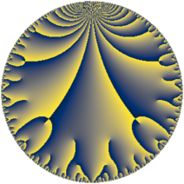# Properties

 Label 289.10.aLevel $289$ Weight $10$ Character orbit 289.a Rep. character $\chi_{289}(1,\cdot)$ Character field $\Q$ Dimension $196$ Newform subspaces $9$ Sturm bound $255$ Trace bound $3$

# Learn more about

## Defining parameters

 Level: $$N$$ $$=$$ $$289 = 17^{2}$$ Weight: $$k$$ $$=$$ $$10$$ Character orbit: $$[\chi]$$ $$=$$ 289.a (trivial) Character field: $$\Q$$ Newform subspaces: $$9$$ Sturm bound: $$255$$ Trace bound: $$3$$ Distinguishing $$T_p$$: $$2$$, $$3$$

## Dimensions

The following table gives the dimensions of various subspaces of $$M_{10}(\Gamma_0(289))$$.

Total New Old
Modular forms 238 211 27
Cusp forms 220 196 24
Eisenstein series 18 15 3

The following table gives the dimensions of the cuspidal new subspaces with specified eigenvalues for the Atkin-Lehner operators and the Fricke involution.

$$17$$Dim.
$$+$$$$96$$
$$-$$$$100$$

## Trace form

 $$196q + 34q^{2} + 148q^{3} + 47958q^{4} - 2842q^{5} + 4142q^{6} + 3814q^{7} + 25602q^{8} + 1167312q^{9} + O(q^{10})$$ $$196q + 34q^{2} + 148q^{3} + 47958q^{4} - 2842q^{5} + 4142q^{6} + 3814q^{7} + 25602q^{8} + 1167312q^{9} - 64898q^{10} - 67500q^{11} + 207850q^{12} + 10812q^{13} - 362552q^{14} + 428808q^{15} + 10244814q^{16} + 1529134q^{18} - 181288q^{19} - 715478q^{20} + 1106564q^{21} + 1100230q^{22} - 3003634q^{23} - 1191342q^{24} + 65253928q^{25} + 15482240q^{26} + 7517332q^{27} + 3145520q^{28} - 4635618q^{29} + 1043358q^{30} + 3715622q^{31} - 7350732q^{32} + 3741768q^{33} + 27291512q^{35} + 214045756q^{36} + 13124210q^{37} + 22147432q^{38} - 43856988q^{39} - 73224598q^{40} - 2288748q^{41} + 43544174q^{42} + 17308040q^{43} - 140019678q^{44} - 121715946q^{45} + 183572956q^{46} - 31987948q^{47} + 304192994q^{48} + 902842108q^{49} + 275535184q^{50} + 1113008q^{52} - 97649496q^{53} - 292953820q^{54} - 143391872q^{55} - 121233936q^{56} - 290228864q^{57} + 172256422q^{58} + 60328920q^{59} + 874804850q^{60} + 127601298q^{61} - 251348744q^{62} + 194130634q^{63} + 2271192898q^{64} + 162954956q^{65} + 154507016q^{66} + 5267144q^{67} + 1640756q^{69} - 1036203924q^{70} - 175871018q^{71} + 466746800q^{72} - 16675856q^{73} - 511462886q^{74} - 765386140q^{75} + 766416100q^{76} - 114390484q^{77} - 1015080612q^{78} - 130907274q^{79} - 220577342q^{80} + 5491482932q^{81} + 63174400q^{82} + 1324473876q^{83} - 1528687596q^{84} - 1419305638q^{86} + 1870991644q^{87} - 114058870q^{88} + 1652886404q^{89} + 4524752134q^{90} + 866519200q^{91} - 3407899844q^{92} - 2809301340q^{93} + 144343396q^{94} + 1750951648q^{95} + 1506989058q^{96} - 2698561500q^{97} + 1512014884q^{98} + 552052864q^{99} + O(q^{100})$$

## Decomposition of $$S_{10}^{\mathrm{new}}(\Gamma_0(289))$$ into newform subspaces

Label Dim. $$A$$ Field CM Traces A-L signs $q$-expansion
$$a_2$$ $$a_3$$ $$a_5$$ $$a_7$$ 17
289.10.a.a $$5$$ $$148.845$$ $$\mathbb{Q}[x]/(x^{5} - \cdots)$$ None $$-33$$ $$236$$ $$-1480$$ $$13202$$ $$+$$ $$q+(-7+\beta _{1})q^{2}+(48-2\beta _{1}-\beta _{4})q^{3}+\cdots$$
289.10.a.b $$7$$ $$148.845$$ $$\mathbb{Q}[x]/(x^{7} - \cdots)$$ None $$-1$$ $$-88$$ $$-1362$$ $$-9388$$ $$+$$ $$q-\beta _{1}q^{2}+(-12-2\beta _{1}+\beta _{4})q^{3}+(341+\cdots)q^{4}+\cdots$$
289.10.a.c $$12$$ $$148.845$$ $$\mathbb{Q}[x]/(x^{12} - \cdots)$$ None $$-30$$ $$0$$ $$0$$ $$0$$ $$+$$ $$q+(-2+\beta _{2})q^{2}-\beta _{1}q^{3}+(154-4\beta _{2}+\cdots)q^{4}+\cdots$$
289.10.a.d $$12$$ $$148.845$$ $$\mathbb{Q}[x]/(x^{12} - \cdots)$$ None $$17$$ $$-74$$ $$-454$$ $$5524$$ $$+$$ $$q+(1+\beta _{1})q^{2}+(-6+\beta _{3})q^{3}+(249+\cdots)q^{4}+\cdots$$
289.10.a.e $$12$$ $$148.845$$ $$\mathbb{Q}[x]/(x^{12} - \cdots)$$ None $$17$$ $$74$$ $$454$$ $$-5524$$ $$-$$ $$q+(1+\beta _{1})q^{2}+(6-\beta _{3})q^{3}+(249-\beta _{1}+\cdots)q^{4}+\cdots$$
289.10.a.f $$24$$ $$148.845$$ None $$0$$ $$0$$ $$0$$ $$0$$ $$+$$
289.10.a.g $$36$$ $$148.845$$ None $$0$$ $$-486$$ $$-3750$$ $$-29040$$ $$+$$
289.10.a.h $$36$$ $$148.845$$ None $$0$$ $$486$$ $$3750$$ $$29040$$ $$-$$
289.10.a.i $$52$$ $$148.845$$ None $$64$$ $$0$$ $$0$$ $$0$$ $$-$$

## Decomposition of $$S_{10}^{\mathrm{old}}(\Gamma_0(289))$$ into lower level spaces

$$S_{10}^{\mathrm{old}}(\Gamma_0(289)) \cong$$ $$S_{10}^{\mathrm{new}}(\Gamma_0(17))$$$$^{\oplus 2}$$